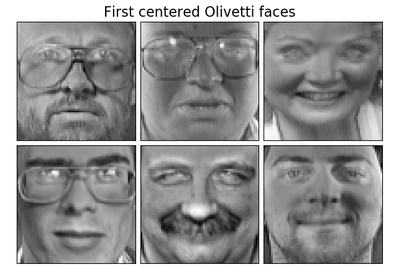# `sklearn.decomposition`.MiniBatchSparsePCA¶

class `sklearn.decomposition.``MiniBatchSparsePCA`(n_components=None, alpha=1, ridge_alpha=0.01, n_iter=100, callback=None, batch_size=3, verbose=False, shuffle=True, n_jobs=1, method=’lars’, random_state=None)[source]

Mini-batch Sparse Principal Components Analysis

Finds the set of sparse components that can optimally reconstruct the data. The amount of sparseness is controllable by the coefficient of the L1 penalty, given by the parameter alpha.

Read more in the User Guide.

Parameters: n_components : int, number of sparse atoms to extract alpha : int, Sparsity controlling parameter. Higher values lead to sparser components. ridge_alpha : float, Amount of ridge shrinkage to apply in order to improve conditioning when calling the transform method. n_iter : int, number of iterations to perform for each mini batch callback : callable or None, optional (default: None) callable that gets invoked every five iterations batch_size : int, the number of features to take in each mini batch verbose : int Controls the verbosity; the higher, the more messages. Defaults to 0. shuffle : boolean, whether to shuffle the data before splitting it in batches n_jobs : int, number of parallel jobs to run, or -1 to autodetect. method : {‘lars’, ‘cd’} lars: uses the least angle regression method to solve the lasso problem (linear_model.lars_path) cd: uses the coordinate descent method to compute the Lasso solution (linear_model.Lasso). Lars will be faster if the estimated components are sparse. random_state : int, RandomState instance or None, optional (default=None) If int, random_state is the seed used by the random number generator; If RandomState instance, random_state is the random number generator; If None, the random number generator is the RandomState instance used by np.random. components_ : array, [n_components, n_features] Sparse components extracted from the data. error_ : array Vector of errors at each iteration. n_iter_ : int Number of iterations run.

Methods

 `fit`(X[, y]) Fit the model from data in X. `fit_transform`(X[, y]) Fit to data, then transform it. `get_params`([deep]) Get parameters for this estimator. `set_params`(**params) Set the parameters of this estimator. `transform`(X[, ridge_alpha]) Least Squares projection of the data onto the sparse components.
`__init__`(n_components=None, alpha=1, ridge_alpha=0.01, n_iter=100, callback=None, batch_size=3, verbose=False, shuffle=True, n_jobs=1, method=’lars’, random_state=None)[source]
`fit`(X, y=None)[source]

Fit the model from data in X.

Parameters: X : array-like, shape (n_samples, n_features) Training vector, where n_samples in the number of samples and n_features is the number of features. y : Ignored. self : object Returns the instance itself.
`fit_transform`(X, y=None, **fit_params)[source]

Fit to data, then transform it.

Fits transformer to X and y with optional parameters fit_params and returns a transformed version of X.

Parameters: X : numpy array of shape [n_samples, n_features] Training set. y : numpy array of shape [n_samples] Target values. X_new : numpy array of shape [n_samples, n_features_new] Transformed array.
`get_params`(deep=True)[source]

Get parameters for this estimator.

Parameters: deep : boolean, optional If True, will return the parameters for this estimator and contained subobjects that are estimators. params : mapping of string to any Parameter names mapped to their values.
`set_params`(**params)[source]

Set the parameters of this estimator.

The method works on simple estimators as well as on nested objects (such as pipelines). The latter have parameters of the form `<component>__<parameter>` so that it’s possible to update each component of a nested object.

Returns: self :
`transform`(X, ridge_alpha=’deprecated’)[source]

Least Squares projection of the data onto the sparse components.

To avoid instability issues in case the system is under-determined, regularization can be applied (Ridge regression) via the ridge_alpha parameter.

Note that Sparse PCA components orthogonality is not enforced as in PCA hence one cannot use a simple linear projection.

Parameters: X : array of shape (n_samples, n_features) Test data to be transformed, must have the same number of features as the data used to train the model. ridge_alpha : float, default: 0.01 Amount of ridge shrinkage to apply in order to improve conditioning. Deprecated since version 0.19: This parameter will be removed in 0.21. Specify `ridge_alpha` in the `SparsePCA` constructor. X_new array, shape (n_samples, n_components) : Transformed data.

## Examples using `sklearn.decomposition.MiniBatchSparsePCA`¶Faces dataset decompositions